# 图算融合加速引擎¶## 背景¶

MindSpore等主流AI计算框架对用户提供的算子通常是从用户可理解、易使用角度进行定义。每个算子承载的计算量不等，计算复杂度也各不相同。但从硬件执行角度看，这种天然的、基于用户角度的算子计算量划分，并不高效，也无法充分发挥硬件资源计算能力。主要体现在：

1. 计算量过大、过复杂的算子，通常很难生成切分较好的高性能算子，从而降低设备利用率；

2. 计算量过小的算子，由于计算无法有效隐藏数据搬移开销，也可能会造成计算的空等时延，从而降低设备利用率；

3. 硬件Device通常为多核、众核结构，当算子shape较小或其它原因引起计算并行度不够时，可能会造成部分核的空闲，从而降低设备利用率。特别是基于专用处理器架构（Domain Specific Architecture，后文简称DSA）的芯片对这些因素更为敏感。如何最大化发挥硬件算力性能的同时使算子也能具备较好的易用性，一直以来是一个很大的挑战。

## 图算融合架构及整体流程¶

1. Composite Expansion：将复合算子展开为基本算子，并构成Composite子图，方便进行后续的跨边界优化和算子拆分；

2. Cross-OP Aggregation：将相邻的基本算子或Composite子图进行聚合，从而构成更大的聚合子图，方便进行后续的跨边界优化和算子拆分；

3. High-Level Optimization：在上面两步得到的聚合子图的基础上，我们可以进行大量的跨边界优化，如代数化简、公共子表达式提取（CSE）等；

4. Kernel Partition：基于计算特征以及融合算子性能，对聚合计算子图进行算子拆分。1. 不同算子之间的跨边界性能优化收益；

2. 通过对整个计算图进行重组拆分，得到最优粒度的融合算子。

## 融合算子加速优化（MindSpore AKG）¶

### 架构及整体流程¶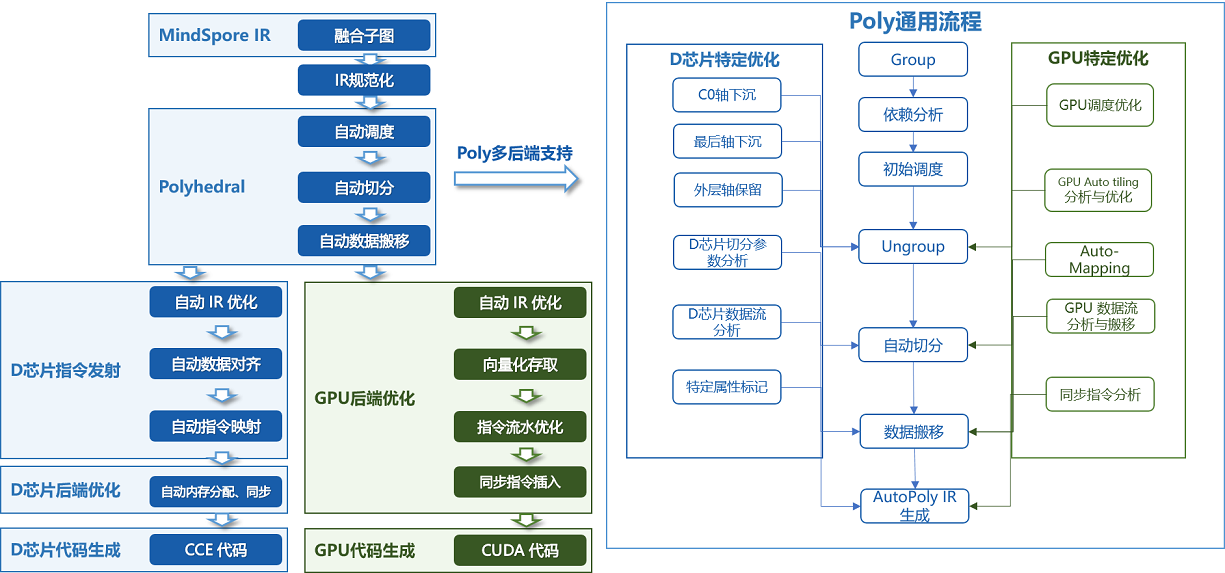MindSpore AKG的整体框架如上图所示：

• IR规范化

• MindSpore AKG的输入为MindSpore图算融合模块优化后的融合子图，通过TVM的Compute / IR Builder / Hybrid 等多种描述方式对子图中的算子进行表达。然后DSL会被转换为 Halide IR（Halide，是常见的用于开发高性能图像处理和Array计算的语言，可作为中间表达解耦算法和优化） 并进行 IR 规范化；

• 完成初步简化和优化后，Halide IR会被转化为Poly模块所需的调度树；

• Poly模块调度优化

• 利用Polyhedral技术中的Pluto调度算法，实现循环的自动融合、自动重排等变换，为融合算子自动生成满足并行性、数据局部性的初始调度；

• 为快速适配不同硬件后端，Poly模块内的优化pass会分为硬件无关的通用优化与硬件相关的特定优化，编译时按照硬件特征拼接组合，实现异构硬件后端的快速适配。自动切分、自动映射以及自动内存提升等pass会根据不同硬件的架构性质给出不同的优化方式；

• 后端优化

• 为了进一步提升算子的性能，我们针对不同硬件后端开发了相应的优化pass，如Ascend后端中实现数据对齐、指令映射，GPU后端中实现向量化存取，插入同步指令等，最终生成相应平台代码。

### 主要特性¶

#### 自动切分（Auto-Tiling）¶

• 什么是切分

切分是一种广泛运用的循环变换的方法，可以改变语句实例访问顺序。如下图代码所示，这个1024 x 1024的循环，每一次循环可以看作是这个二维空间上的一个点的访问，对这个循环进行4 x 4的切分能够改变循环点的访问顺序，使得从原先的遍历大矩阵变成多次遍历4 x 4的小矩阵。• 切分的价值和挑战

将数据进行分块和内存映射，可以使用更小更快的缓存来访问数据。当计算数据量大于缓存空间的时候，就需要将原来的数据进行分块后存储到缓存上，以此来适应目标架构的硬件特征。要寻找一个好的切分，开发者需要对硬件的内存层级和代码逻辑有所了解，能够分析出有哪些数据逻辑上被复用，需要被放进缓存，甚至还需要对硬件的缓存机制有所了解（例如CPU），对硬件的并行机制有所了解（例如GPU），这样的切分才能够提升硬件资源利用率，提高代码的性能。

• MindSpore AKG的自动切分方案

MindSpore AKG中提供了Auto-Tiling模块，其主要流程包括：

1. 对输入分析建模。Auto-Tiling借助Poly模块，将输入算子的逻辑转化为调度树，轴空间提取模型对调度树做分析，提取出可切分调度的总体轴空间；

2. 对轴空间进行剪枝。Auto-Tiling使用两个约束生成模型对算子逻辑和硬件信息分别进行分析，生成对应的算子约束和硬件约束，其中这些约束根据影响算子的范围又会被分为强弱约束，它们作用在轴空间上，将轴空间缩小，即可获得一个约束轴空间；

3. 轴空间求解。Auto-Tiling会根据算子和硬件信息生成不同的目标优化函数，求解模型基于目标优化函数，在约束轴空间上能够找到唯一一个解，生成最终的切分调度配置。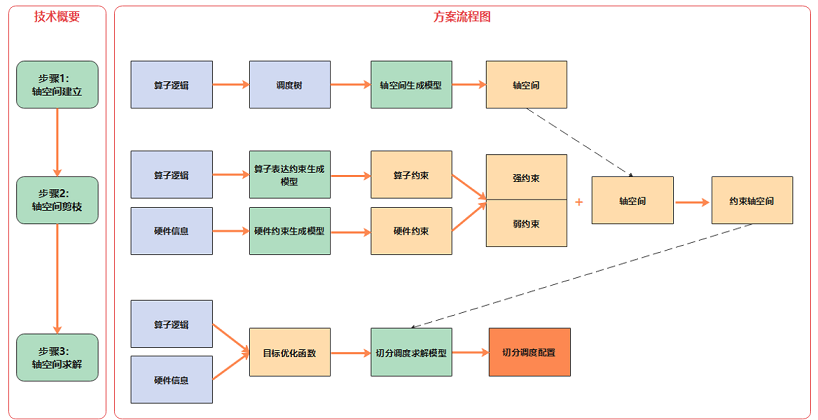#### 自动映射（Auto-Mapping）¶

• 减少代码复杂度

如下图所示，shape为8 * 12的算子，Auto-Tiling会尽量采取(a)中的分块方式，来减少(b)中蓝字所示的循环边界判断；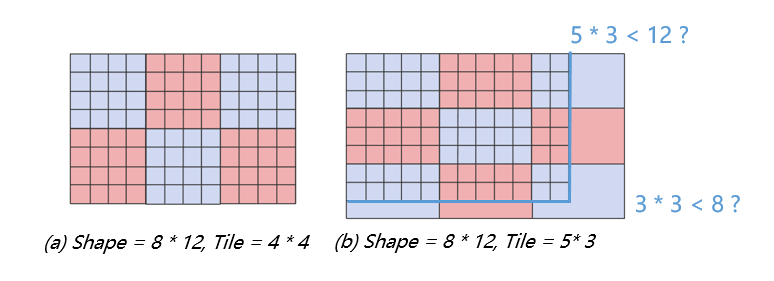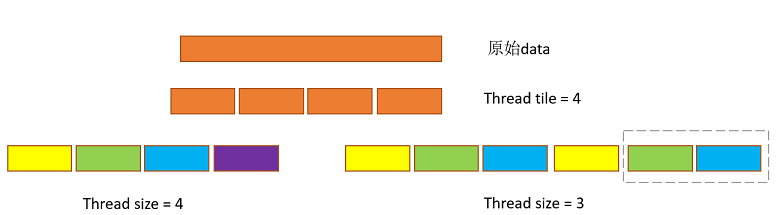#### 数据搬移¶

MindSpore AKG基于Polyhedral技术来实现基于多层Buffer结构的DMA数据流识别与生成。自动数据搬移能够通过分析数据流，给出数据应该放置到什么缓冲区，以及数据在缓冲区之间搬移的顺序，进一步优化算子性能。

1. 依次遍历算子输入的Halide IR，识别每个compute节点的类型，并根据不同类型分析每条语句需使用到的计算模块；

2. 根据目标芯片的片上缓存模型和具体芯片参数，对片上的高速存储Buffer进行分类；

3. 基于每条语句使用的计算单元，以及变量间的数据依赖关系（每个计算单元，会从不同的片上Buffer读入数据，并将计算结果写回不同的片上缓存，其依赖关系可以分为读后写WAR，写后读RAW，写后写WAW三种），生成算子数据流信息；

4. 对于可融合的场景，将算子的数据流进行优化，得到完整的算子数据流信息；

5. 输出包含算子完整数据流信息的Halide IR。### 算子优化方案¶

• 规约计算 Reduction

规约计算，即对Tensor的选定维度或所有维度做累积操作，常见的算子有Sum、ReduceMax/Min、ReduceAnd/Or等。 大shape的Reduction场景通常分为两步：

1. 将数据分成小块，分别对各小块进行规约；

2. 对每个小块的规约结果再次进行规约得到最终结果。

MindSpore AKG通过自动轴融合+多面体调度优化+AKG-Reduce模板库的方式对reduction操作进行优化，并通过原子加的方式，将两步reduce实现成一个kernel。流程如上图所示：

1. 算子表示：首先解析算子表达，在IR规范化的阶段，进行规约轴和非规约轴的识别与分离，并通过自动轴融合，将两类轴分别融合成两根轴，同时记录相关信息如Tensor shape、规约方向等；

2. Poly模块：利用Pluto算法进行调度变换，通过分析之前记录的信息，计算得到最佳切分参数，并进行到共享内存、寄存器的数据搬移；

3. 指令发射与代码生成：从调度树到Halide IR的发射阶段，插入接口调用语句并在代码生成阶段调用。调用AkgReduce模板库，实现高性能的线程块内累加；调用AkgAtomicReturn原子加接口，将各线程块的中间结果累加得到最终结果，保证计算正确性。

• 矩阵乘 GEMM卷积 Convolution

MindSpore AKG利用GPU的Tensor Core硬件计算单元并结合多面体编译调度优化和高性能内联PTX库，对混合精度场景下的矩阵乘计算进行加速。

在此基础上，MindSpore AKG使用Implicit GEMM方法来处理混合精度的卷积计算。卷积的两个四维的输入矩阵，会在从全局内存搬移到共享内存的过程中，被转换成二维矩阵，进而转化为矩阵乘计算进行优化。这种方法可以解决Image to Column带来的数据冗余（Image to Column是常见的卷积优化方法，简称为Im2col，即将每个feature map转换为一个连续的column，转换后的矩阵会占据更多的全局内存）。

以卷积计算的优化为例，流程如下：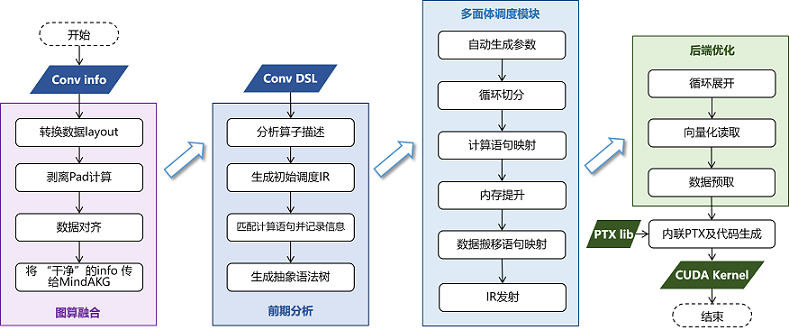1. 分析算子DSL和Halide IR，解析GEMM表达式并记录矩阵的数据类型、shape、排布方式等信息；卷积算子的shape更复杂（通常是NHWC四个维度），在这一步MindSpore AKG会将它的四个维度抽象出来，关联到与矩阵乘对应的两个虚拟轴上；

2. 在Poly模块中进行针对性调度优化，包含多次切分、Warp层级映射，多层级内存提升（共享内存上进行数据复用，寄存器上使用TensorCore进行高速的乘加运算）等；对于卷积算子，进行多次切分以及计算切分参数时，也需要考虑多出来的两个维度H、W；

3. 基于Halide IR执行后端优化pass，包括数据预取——节省搬运与计算间的等待时间、数据补齐与重排——消除bank conflicts、向量化指令——提升数据加载和写入的效率等；

4. 调用akg::wmma高性能接口（包含PTX层级的更细粒度的优化），生成最终的CUDA Kernel。

用户和开发者可以通过运行MindSpore AKG的测试用例了解融合算子或复杂算子的优化流程，以卷积算子的相关代码为例：

四维卷积（不含Pad操作）的计算公式如下，其中$$N = 32, H = W = 28, Co = 128, Ci = 64, Hk = Hw = 5$$

$Output(n, h, w, o)=\sum_{c=1}^{Ci} \sum_{rh=1}^{Hk} \sum_{rw=1}^{Wk} (Image(n, h+rh, w+rw, c)*Filter(o, rh, rw, c))$

根据其计算公式，可以使用tvm.compute编写出算子DSL：

n, in_h, in_w, in_c = data.shape
out_c, k_h, k_w, in_c = weight.shape
_, _, s_h, s_w = stride
o_h = (in_h - k_h) // s_h + 1
o_w = (in_w - k_w) // s_w + 1
rc = tvm.reduce_axis((0, in_c), name="rc")
rh = tvm.reduce_axis((0, k_h), name="rh")
rw = tvm.reduce_axis((0, k_w), name="rw")
output = tvm.compute(
(n, o_h, o_w, out_c),
lambda n, h, w, o: tvm.sum(
data[n, (h * s_h + rh), (w * s_w + rw), rc]
* weight[o, rh, rw, rc],
axis=[rc, rh, rw]),
name=output_name
)
return output


生成出的初始调度如下，包含7个for循环，计算效率较低：

// attr [compute(out, 0x55c9185ce710)] realize_scope = ""
realize out<float16>([0, 32], [0, 28], [0, 28], [0, 128]) {
produce out {
for (n, 0, 32) {
for (h, 0, 28) {
for (w, 0, 28) {
for (o, 0, 128) {
out(n, h, w, o) = 0h
for (rc, 0, 64) {
for (rh, 0, 5) {
for (rw, 0, 5) {
// attr [[iter_var(rc, range(min=0, ext=64)), iter_var(rh, range(min=0, ext=5)), iter_var(rw, range(min=0, ext=5))]] reduce_update = ""
out(n, h, w, o) = (out(n, h, w, o) + (input_1(n, (h + rh), (w + rw), rc)*input_2(o, rh, rw, rc)))
}
}
}
}
}
}
}
}
}


经过Poly模块调度优化、多个后端优化pass和代码生成后，大大提高了算子的程序并行性和数据局部性，得到的最终在GPU上执行的CUDA kernel如下：

// 引入akg_mma_lib高性能库
#include "akg_mma_lib/wmma.hpp"
extern "C" __global__ void conv_tc_auto_float16_32_32_32_64_float16_128_5_5_64_1_1_0_0_0_0_1_1_float16_kernel0( half* __restrict__ input_1,  half* __restrict__ input_2,  half* __restrict__ out) {
// 缓冲区分配
akg::wmma::fragment<nvcuda::wmma::accumulator, 16, 16, 8, float> out_local;
half input_2_shared_transfer;
__shared__ half input_2_shared;
half input_1_shared_transfer;
akg::wmma::fragment<nvcuda::wmma::matrix_b, 16, 16, 8, half, nvcuda::wmma::col_major> input_2_local;
akg::wmma::fragment<nvcuda::wmma::matrix_a, 16, 16, 8, half, nvcuda::wmma::row_major> input_1_local;
#pragma unroll
for (int cc5 = 0; cc5 < 5; ++cc5) {
// 将本次计算要用的数据从全局内存预先加载到共享内存中
// 用float4指针进行向量化读取
#pragma unroll
for (int cc7 = 0; cc7 < 4; ++cc7) {
((float4*)input_2_shared_transfer)[((cc7 * 8) + 0) / 8] = ((float4*)input_2)[(((((cc7 * 51200) + ((((int)threadIdx.x) / 8) * 1600)) + (cc5 * 320)) + ((((int)threadIdx.x) % 8) * 8)) + 0) / 8];
}
#pragma unroll
for (int cc71 = 0; cc71 < 4; ++cc71) {
((float4*)input_2_shared)[(((((cc71 * 2176) + ((((int)threadIdx.x) / 128) * 1088)) + ((((int)threadIdx.x) % 8) * 136)) + (((((int)threadIdx.x) % 128) / 8) * 8)) + 0) / 8] = ((float4*)input_2_shared_transfer)[((cc71 * 8) + 0) / 8];
}
#pragma unroll
for (int cc72 = 0; cc72 < 2; ++cc72) {
((float4*)input_1_shared_transfer)[((cc72 * 8) + 0) / 8] = ((float4*)input_1)[(((((((cc72 * 1048576) + ((((int)threadIdx.x) / 16) * 65536)) + ((((int)blockIdx.y) / 14) * 2048)) + (cc5 * 2048)) + ((((int)blockIdx.y) % 14) * 128)) + ((((int)threadIdx.x) % 16) * 8)) + 0) / 8];
}
#pragma unroll
for (int cc73 = 0; cc73 < 2; ++cc73) {
((float4*)input_2_shared)[(((((cc73 * 2176) + ((((int)threadIdx.x) % 16) * 136)) + ((((int)threadIdx.x) / 16) * 8)) + 0) + 8704) / 8] = ((float4*)input_1_shared_transfer)[((cc73 * 8) + 0) / 8];
}
#pragma unroll
for (int cc6_outer = 0; cc6_outer < 4; ++cc6_outer) {
// 将下次计算要用的数据从全局内存预先加载到寄存器中
#pragma unroll
for (int cc74 = 0; cc74 < 4; ++cc74) {
((float4*)input_2_shared_transfer)[((cc74 * 8) + 0) / 8] = ((float4*)input_2)[(((((((cc74 * 51200) + ((((int)threadIdx.x) / 8) * 1600)) + (cc5 * 320)) + (cc6_outer * 64)) + ((((int)threadIdx.x) % 8) * 8)) + 0) + 64) / 8];
}
#pragma unroll
for (int cc75 = 0; cc75 < 2; ++cc75) {
((float4*)input_1_shared_transfer)[((cc75 * 8) + 0) / 8] = ((float4*)input_1)[(((((((((cc75 * 1048576) + ((((int)threadIdx.x) / 16) * 65536)) + ((((int)blockIdx.y) / 14) * 2048)) + (cc5 * 2048)) + ((((int)blockIdx.y) % 14) * 128)) + (cc6_outer * 64)) + ((((int)threadIdx.x) % 16) * 8)) + 0) + 64) / 8];
}
// 调用高性能接口进行数据搬移、初始化和mma计算
#pragma unroll
for (int cc11 = 0; cc11 < 8; ++cc11) {
#pragma unroll
for (int cc123 = 0; cc123 < 2; ++cc123) {
(void)akg::wmma::load_matrix_sync(input_2_local[cc123], &(input_2_shared[((((((int)threadIdx.x) / 64) * 2176) + (cc123 * 1088)) + (cc11 * 136))]), 8);
}
#pragma unroll
for (int cc124 = 0; cc124 < 2; ++cc124) {
(void)akg::wmma::load_matrix_sync(input_1_local[cc124], &(input_2_shared[((((((((int)threadIdx.x) % 64) / 32) * 2176) + (cc124 * 1088)) + (cc11 * 136)) + 8704)]), 8);
}
#pragma unroll
for (int cc21 = 0; cc21 < 2; ++cc21) {
#pragma unroll
for (int cc22 = 0; cc22 < 2; ++cc22) {
if (((cc5 == 0) && (cc6_outer == 0)) && (cc11 == 0)) {
(void)akg::wmma::fill_fragment(out_local[((cc21 * 2) + cc22)], 0.000000e+00f);
}
(void)akg::wmma::mma_sync(out_local[((cc21 * 2) + cc22)], input_1_local[cc21], input_2_local[cc22], out_local[((cc21 * 2) + cc22)]);
}
}
}
//  将下次计算要用的数据从寄存器搬到共享内存中
#pragma unroll
for (int cc76 = 0; cc76 < 4; ++cc76) {
((float4*)input_2_shared)[(((((cc76 * 2176) + ((((int)threadIdx.x) / 128) * 1088)) + ((((int)threadIdx.x) % 8) * 136)) + (((((int)threadIdx.x) % 128) / 8) * 8)) + 0) / 8] = ((float4*)input_2_shared_transfer)[((cc76 * 8) + 0) / 8];
}
#pragma unroll
for (int cc77 = 0; cc77 < 2; ++cc77) {
((float4*)input_2_shared)[(((((cc77 * 2176) + ((((int)threadIdx.x) % 16) * 136)) + ((((int)threadIdx.x) / 16) * 8)) + 0) + 8704) / 8] = ((float4*)input_1_shared_transfer)[((cc77 * 8) + 0) / 8];
}
}
#pragma unroll
for (int cc111 = 0; cc111 < 8; ++cc111) {
#pragma unroll
for (int cc126 = 0; cc126 < 2; ++cc126) {
(void)akg::wmma::load_matrix_sync(input_2_local[cc126], &(input_2_shared[((((((int)threadIdx.x) / 64) * 2176) + (cc126 * 1088)) + (cc111 * 136))]), 8);
}
#pragma unroll
for (int cc127 = 0; cc127 < 2; ++cc127) {
(void)akg::wmma::load_matrix_sync(input_1_local[cc127], &(input_2_shared[((((((((int)threadIdx.x) % 64) / 32) * 2176) + (cc127 * 1088)) + (cc111 * 136)) + 8704)]), 8);
}
#pragma unroll
for (int cc211 = 0; cc211 < 2; ++cc211) {
#pragma unroll
for (int cc221 = 0; cc221 < 2; ++cc221) {
(void)akg::wmma::mma_sync(out_local[((cc211 * 2) + cc221)], input_1_local[cc211], input_2_local[cc221], out_local[((cc211 * 2) + cc221)]);
}
}
}
}
#pragma unroll
for (int cc4 = 0; cc4 < 2; ++cc4) {
#pragma unroll
for (int cc6 = 0; cc6 < 2; ++cc6) {
(void)akg::wmma::store_matrix_sync(&(input_2_shared[((((((((int)threadIdx.x) % 64) / 32) * 4352) + (cc4 * 136)) + ((((int)threadIdx.x) / 64) * 32)) + (cc6 * 16))]), out_local[((cc4 * 2) + cc6)], 272, nvcuda::wmma::mem_row_major);
}
}
// 将计算结果搬出到全局内存的输出缓冲区